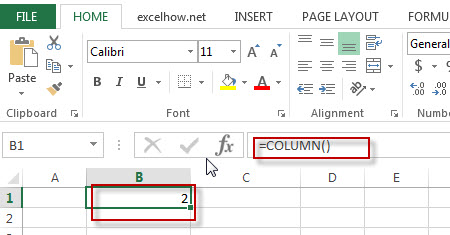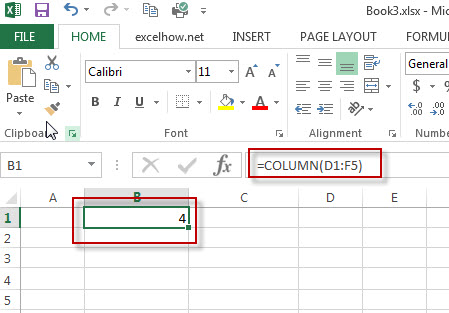# Excel Column Function

This post will guide you how to use Excel COLUMN function with syntax and examples in Microsoft excel.

### Description

The Excel COLUMN function returns the first column number of the given cell reference.

The COLUMN function is a build-in function in Microsoft Excel and it is categorized as a Lookup and Reference Function.

The COLUMN function is available in Excel 2016, Excel 2013, Excel 2010, Excel 2007, Excel 2003, Excel XP, Excel 2000, Excel 2011 for Mac.

### Syntax

The syntax of the COLUMN function is as below:

=COLUMN ([reference])

Where the COLUMN function arguments is:
Reference -This is an optional argument.  A reference to a cell or a range of cells for which you want to get the first column number.
Note: If the Array argument is omitted, the Excel COLUMN function will return the column number of the cell that the function is entered in.

### Example

The below examples will show you how to use Excel COLUMN Lookup and Reference Function to return the column number of a cell reference.

#1 To get the number of column in B1 Cell, just using the following excel formula: =COLUMN ( )#2 To get the number of column in the reference D1:F5, just using the following excel formula: =COLUMN(D1:F5)### More Excel Column Function Examples

• VLOOKUP Return Multiple Values Horizontally
You can create a complex array formula based on the INDEX function, the SMALL function, the IF function, the ROW function and the COLUMN function to vlookup a value and then return multiple corresponding values horizontally in Excel.…
• Sum Every Nth Row or Column
If you want to sum every nth rows in Excel, you can create an Excel Array formula based on the SUM function, the MOD function and the ROW function..….